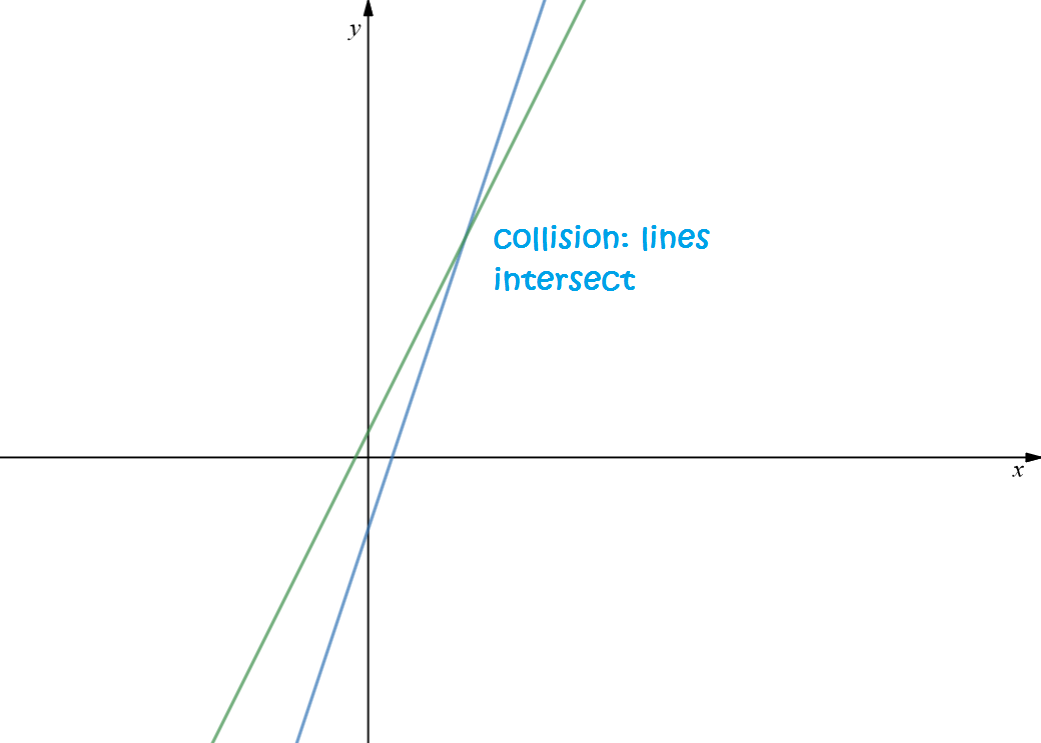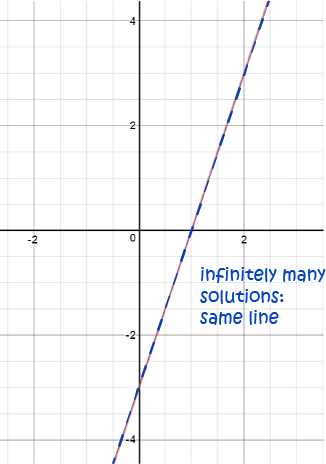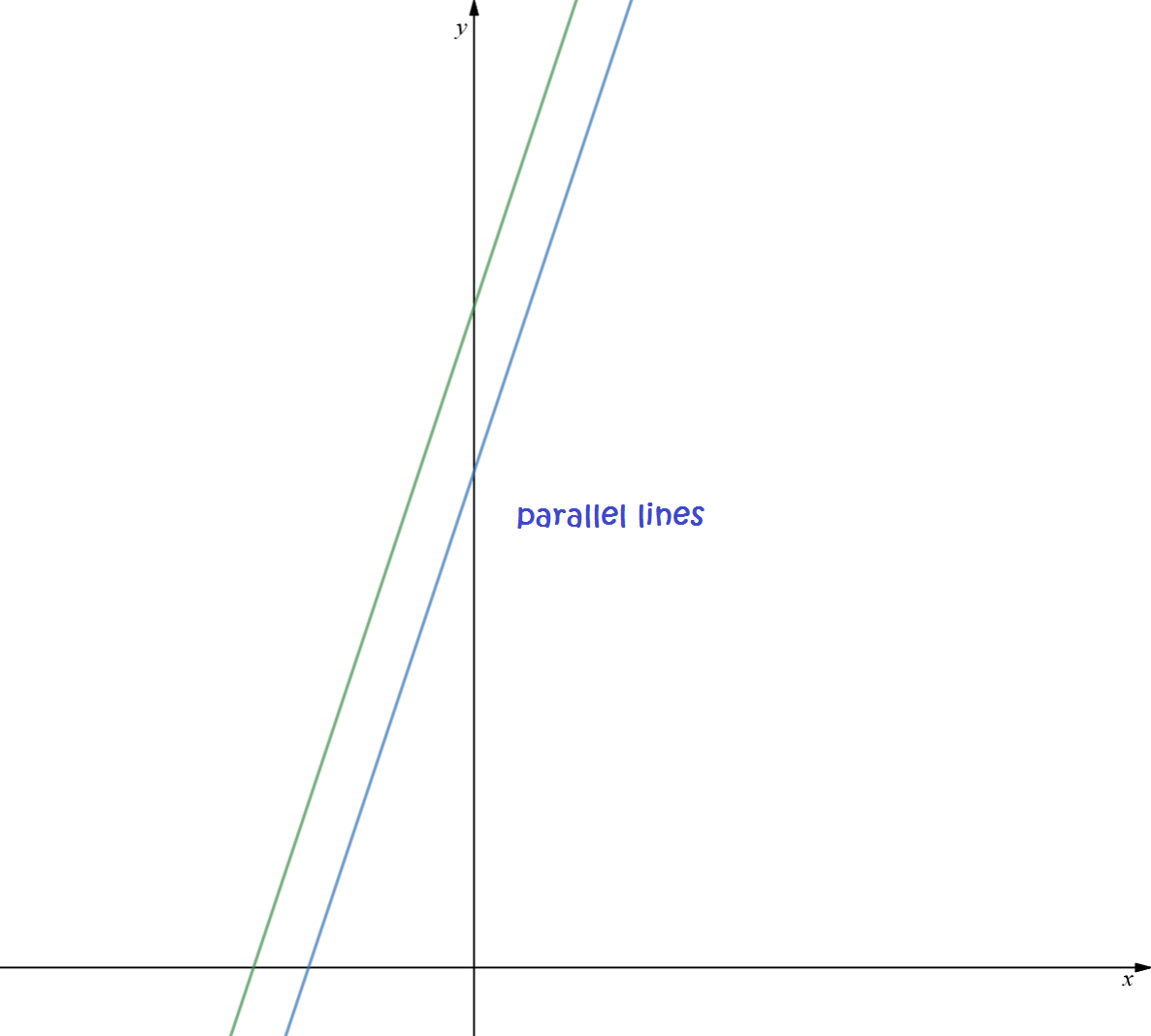# Solving Systems of Linear Equations: Elimination

Elimination means "getting rid" of something. So, when we solve a system of equations by elimination, we get rid of variables, one by one.

## The Method

There are a number of operations we can perform on a system of equations without changing the solutions. They are:

• Multiply both sides of one of the equations by a constant.
• Add a multiple of one equation to another.
• Swap the positions of two or more equations.

When we solve a system of equations by elimination, we apply these operations to get rid of the variables one by one until we're left with only one variable.

Let's see how we add two equations together. We'll try the equations $3x + 4y = 5$ and $2x + 5y = 7$:

\begin{align*} 3x + 4y &= 5\\ 2x + 5y &= 7\\ \hline 5x + 9y &= 12 \end{align*}
Can you see that the terms in $x$ were added together, the terms in $y$ were added together and the right hand sides of the equations were added together? This doesn't change the solutions because we are just adding the same thing to both sides. That's what "=" means!

Let's try multiplying an equation by a constant. We'll multiply the equation $3x + 4y = 5$ by 4. All we do is multiply each term on both sides of the equation by $4$, so

\begin{align*} 3x + 4y &= 5 \\ (3x) \times 4 + (4y) \times 4 &= 5 \times 4\\ 12x + 16 y &= 20 \end{align*}
Again, we haven't changed the solutions because we've done the same operation to both sides of the equation.

Let's see the technique in action on some examples with two equations in two variables:

### Example 1

Find the solutions (if they exist) of the system of linear equations

\begin{align*} 3x - y &= 3\\ 2x - y &= -1 \end{align*}

Both of these equations include a term of $-y$, so the easiest thing to do is subtract the second one from the first one like this:

\begin{align*} 3x - y &= 3 \;\;\;\;\;\;\; -\\ 2x - y &= -1\\ \hline (3x - 2x) - (y - (-y)) &= 3 - (-1)\\ \end{align*}
which gives $x = 4$ after simplification.

Now substitute $x =4$ into one of the equations (doesn't matter which) to work out what $y$ is. Let's use equation 2:

\begin{align*} 2x - y &= -1\\ 2(4) - y &= -1\\ -y &= -9\\ y &= 9 \end{align*}
So, the solution is $x = 4$ and $y = 9$, which you can see as the point of intersection on the graph below:Note: Because there is a solution, the equations are consistent. However, you should always check that your solution works for both equations. I'll leave that up to you.

Let's try another example.

### Example 2

Find the solutions (if they exist) of the system of linear equations

\begin{align*} 3x - y &= 3\\ 9x - 3y &= 9 \end{align*}

Let's get rid of the $x$s. The second equation as a term of $9x$ and the first equation has a term of $3x$. Multiply the first equation by 3 and subtract the result from the second one like this:

\begin{align*} 9x - 3y &= 9 \;\;\;\;\;\;\; - \\ 3(3x - y) &= 3(3)\\ \hline 9x - 3y &= 9\;\;\;\;\;\;\; - \\ 9x - 3y &= 9\\ \hline 0 - 0 &= 0 \end{align*}
which works, regardless of what the values of $x$ and $y$ are. So, the equations have infinitely many solutions. They are dependent, and represent the same straight line! Let's see what this looks like on a graph:Let's see what happens if there are no solutions.

### Example 3

Solve the system of linear equations:

\begin{align*} 3x - y &= -4\\ 3x - y &= -3\\ \end{align*}

Let's eliminate the $x$s (exterminate, exterminate!). Both equations include a term of $3x$, so subtract the second equation from the first:

\begin{align*} 3x - y &= -4 \;\;\;\;\;\;\; -\\ 3x - y &= -3\\ \hline 0 - 0 &= -1\\ \end{align*}
Uh-oh! That got rid of the $y$s as well, but it didn't get rid of the $-1$ on the right hand side. We've ended up with a nonsensical equation of $0 = -1$. Don't panic! This just means that our system of linear equations has no solution. The two lines are parallel. Here's what they look like:## Solving Systems with 3 Equations By Elimination

This technique will generalise to any number of equations in any number of unknowns. I'm going to give you a set of steps that will always work. When you get good at solving systems of equations by elimination, you might decide to adapt them sometimes to avoid making mistakes: for example you might like to get rid of $y$ first instead of $x$ because the arithmetic is easier. Here are the steps:

1. Eliminate the variables in alphabetical order:
• First eliminate the $x$s from equations 2 and 3
• Next eliminate the $y$s from equation 3
This will give you a system that looks like this:
\begin{align*} a_1 x + b_1 y + c_1 &= d_1\\ \;\;\;\;\;\;b_4 y + c_4z &= d_4 \mbox{ these coefficients have been changed by the elimination operations}\\ \;\;\;\;\;\;\;\;\;\;c_5z &= d_5 \mbox{ these coefficients have been changed by the elimination operations}\\ \end{align*}
2. Back-substitute: start by working out $z$ from equation 3, then use your value of $z$ to find $y$ using equation 2, then use your values of $y$ and $z$ to find $x$ using equation 1. We work from the bottom up.

As it's always easier to see these things in action on an example, let's do just that!.

### Example 4

Solve the system of equations

\begin{align*} x\;\;\;\; &&- &z &= &4\\ &- 2y & + &z &= &8\\ 3x\;\;\;\;& + y& + &4z &= &83 \end{align*}

Step 1: get rid of the $x$s from equations 2 and 3. Equation 2 contains no $x$, so we can leave it, but equation 3 contains a term of $3x$. Subtract 3 lots of equation 1 from equation 3 to yield a new version of equation 3:

\begin{align*} 3x\;\;\;\;& + y& + &4z &= &83 \;\;\;\;\;\;-\\ 3 \times (x\;\;\;\;\;\;&& - &z) &= & 3 \times 4\\ \hline 3x\;\;\;\;& + y& + &4z &= &83 \;\;\;\;\;\;-\\ 3x\;\;\;\;& + 0& - &3z &= &12 \;\;\;\;\;\;\\ \hline \;\;\;\;\;& y & + & 7z &= &71 \end{align*}

Our system of equations now looks like this:

\begin{align*} x\;\;\;\; &&- &z &= &4\\ &- 2y & + &z &= &8\\ \;\;\;\;\;\;\;\;& y& + &7z &= &71 \end{align*}

Step 2: To make the arithmetic easier, swap equations 2 and 3 to yield:

\begin{align*} x\;\;\;\; &&- &z &= &4\\ &y& + &7z &= &71\\ &-2y & + &z &= &8 \end{align*}

Step 3: Get rid of the $y$s from equation 3 by adding two lots of the second equation to it:

\begin{align*} 2(y& + &7z) &= &2(71)\;\;\;\;\;\; +\\ -2y& + &z &= &8\;\;\;\;\;\;\\ \hline 2y& + &14z &=&142\;\;\;\;\;\;+\\ -2y &+ &z &=&8\;\;\;\;\;\\ \hline 0 &+&15z &=&150 \end{align*}

Now it's time for back-substitution:

Step 4: Solve equation 3 for $z$.

\begin{align*} 15 z &=150\\ z &= 10 \mbox{ after dividing both sides by 10} \end{align*}

Step 5: Plug this value for $z$ into equation 2 and solve for $y$:

\begin{align*} y + 7z &= 71\\ y + 7(10) &= 71\\ y + 70 &= 71\\ y &= 1 \end{align*}

Step 6: Finally, plug the values for $y$ and $z$ into equation 1 and solve for $x$:

\begin{align*} x - z &= 4\\ x - 10 &= 4\\ x &= 14\\ \end{align*}

So the system of equations has a unique solution: $x = 14$, $y = 1$ and $z = 10$. This corresponds to the point $(14,1,10)$. You should plug these values into the three equations to make sure they work for all three equations.

### Conclusion

The solutions of a system of linear equations need to work for all equations in the system simultaneously. For this reason, systems of linear equations are often called simultaneous equations.

The elimination method is more efficient for larger numbers of equations and larger numbers of variables than the substitution method. A computer can also be programmed to solve the equations using this technique.

With practice, you'll get a lot quicker at solving systems of linear equations. You'll also start to be able to spot some short cuts which will make the arithmetic easier.

### Description

coordinate geometry is a branch of geometry where the position of the points on the plane is defined with the help of an ordered pair of numbers also known as coordinates. In this tutorial series, you will learn about vast range of topics such as Cartesian Coordinates, Midpoint of a Line Segment etc

### Audience

year 10 or higher, several chapters suitable for Year 8+ students.

### Learning Objectives

Explore topics related to Coordinates Geometry

Author: Subject Coach
You must be logged in as Student to ask a Question.